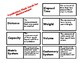# Math Vocabulary Unit - MeasurementSubject
Resource Type
File Type

PDF

(744 KB|10 pages)
Standards
Also included in:
1. Students need to know and understand math vocabulary for more than just the ability to pass their math test. Students also need math vocabulary in order to solve word problems, justify their answers and have good math conversations. This mathematical terms bundle comes with 9 different math vocabu
\$45.00
\$36.00
Save \$9.00
• Product Description
• Standards
Students need to know and understand math vocabulary for more than just the ability to pass their math test. Students also need math vocabulary in order to solve word problems, justify their answers and have good math conversations.

This mathematical terms unit includes: Word Wall Cards, a Vocabulary Pre/Post Quiz, Vocabulary Flash Cards and Crossword Puzzle Practice. These elements come with a list of suggested classroom use.

For the measurement vocabulary packet, the vocabulary words provided are: distance, capacity, weight, volume, elapsed time, metric system, customary system, centimeter, inches, gram, ounce, meter, foot, liter and cup.

See exactly what you're receiving when you download this file, by clicking on the green PREVIEW button.

This packet is also included in my Math Vocabulary Bundle which includes:

Problem Solving

Number Sense and Place Value

Multiplication and Division

Fractions and Decimals

Geometrical Shapes

Geometrical Lines and Angles

Measurement

Data and Graphing

Created by Heidi Raki of Raki's Rad Resources.
Attend to precision. Mathematically proficient students try to communicate precisely to others. They try to use clear definitions in discussion with others and in their own reasoning. They state the meaning of the symbols they choose, including using the equal sign consistently and appropriately. They are careful about specifying units of measure, and labeling axes to clarify the correspondence with quantities in a problem. They calculate accurately and efficiently, express numerical answers with a degree of precision appropriate for the problem context. In the elementary grades, students give carefully formulated explanations to each other. By the time they reach high school they have learned to examine claims and make explicit use of definitions.
Recognize volume as an attribute of solid figures and understand concepts of volume measurement.
Convert among different-sized standard measurement units within a given measurement system (e.g., convert 5 cm to 0.05 m), and use these conversions in solving multi-step, real world problems.
Use the four operations to solve word problems involving distances, intervals of time, liquid volumes, masses of objects, and money, including problems involving simple fractions or decimals, and problems that require expressing measurements given in a larger unit in terms of a smaller unit. Represent measurement quantities using diagrams such as number line diagrams that feature a measurement scale.
Know relative sizes of measurement units within one system of units including km, m, cm; kg, g; lb, oz.; l, ml; hr, min, sec. Within a single system of measurement, express measurements in a larger unit in terms of a smaller unit. Record measurement equivalents in a two-column table. For example, know that 1 ft is 12 times as long as 1 in. Express the length of a 4 ft snake as 48 in. Generate a conversion table for feet and inches listing the number pairs (1, 12), (2, 24), (3, 36),...
Total Pages
10 pages
Included
Teaching Duration
1 month
Report this Resource to TpT
Reported resources will be reviewed by our team. Report this resource to let us know if this resource violates TpT’s content guidelines.